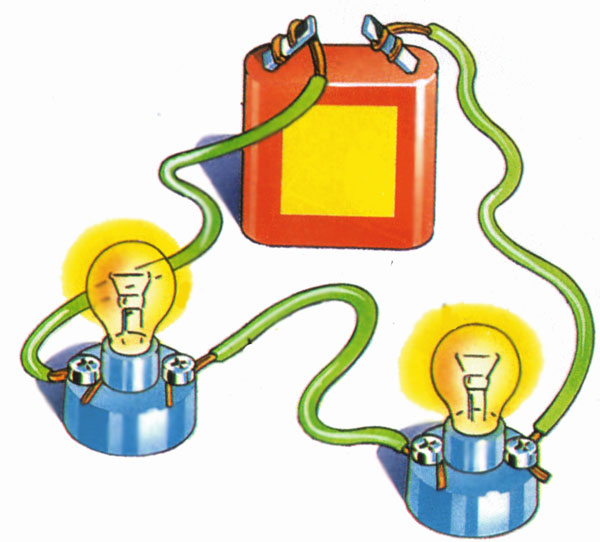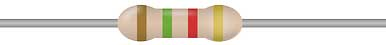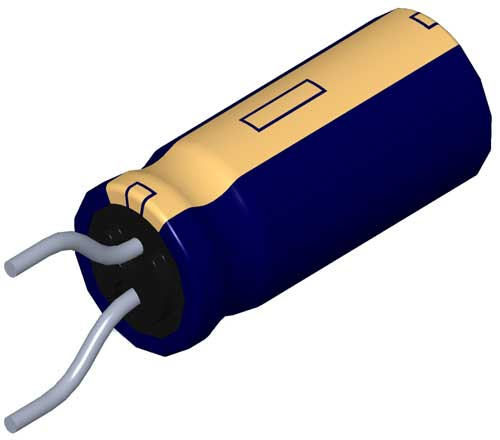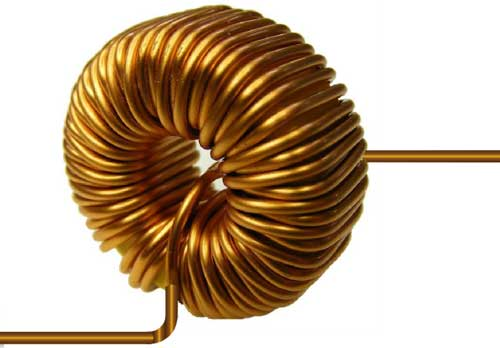Electric circuits are used to provide a path to electric current. They commonly use three basic electrical components, which are resistors, capacitors, and insulators. They affect the electric current and changes the behavior of the circuit.

ResistorA resistor is an electrical component, which has a certain amount of resistance. Resistance is the measure of the opposition to electric current. The more resistance a resistor has, the lesser current will flow through it. Resistance is measured in the unit called ohms, and it is abbreviated with the Greek symbol Ω (omega).

Resistance is found by the using the ohm’s law. Which says that resistance in ohms is equal to voltage divided by current.

Resistance = Voltage/Current

OR

R = V/I

Resistors are represented by a zigzag line in an electric circuit, and the letter ‘R’ is used in the equations.

CapacitorElectric current is the flow of electric charges, much like the flow of water. As water can be stored, charges can also be stored. The electrical component that stores the electric charges is called capacitor. Capacitor’s ability to store the charge is called capacitance, which is measured in ‘Farads’ and abbreviated as ‘F’. The more capacitance a capacitor has, the more electric charge can be stored in it. Capacitors are represented by the two parallel plates in an electric circuit.

The capacitance of a capacitor is found by the formula:

Capacitance = Charge Stored/Voltage

OR

C = q/V ;              q = stored charge

V = Voltage of the capacitor

InductorThe opposition to change in current is called inductance, and an electrical component which has inductance is called inductor. Inductors are like the short circuits to the DC current, because DC current doesn’t change the magnitude. But, Inductors provide an opposition like a resistor to the AC current. Because AC current constantly change the magnitude and the polarity.